# ML Aggarwal Solutions for Class 7 Maths Chapter 8 Algebraic Expressions

ML Aggarwal Solutions for Class 7 Maths Chapter 8 Algebraic Expressions are given here. These Solutions are most valuable, that assist students in learning complex and challenging topics. By studying the questions of ML Aggarwal Solutions for Class 7 Maths Chapter 8, students can enhance their execution abilities and operate on their strengths and weaknesses. It makes the students feel confident while answering the question paper.

Chapter 8 – Algebraic Expressions. Expressions are defined as, numbers, symbols and operators (such as +. – , × and ÷) grouped together that show the value of something. This chapter contains the topics related to terms of an expression, like and unlike terms, monomials, binomials, trinomials and polynomials, addition and subtraction of algebraic expressions.

## Download the PDF of ML Aggarwal Solutions for Class 7 Maths Chapter 8 Algebraic Expressions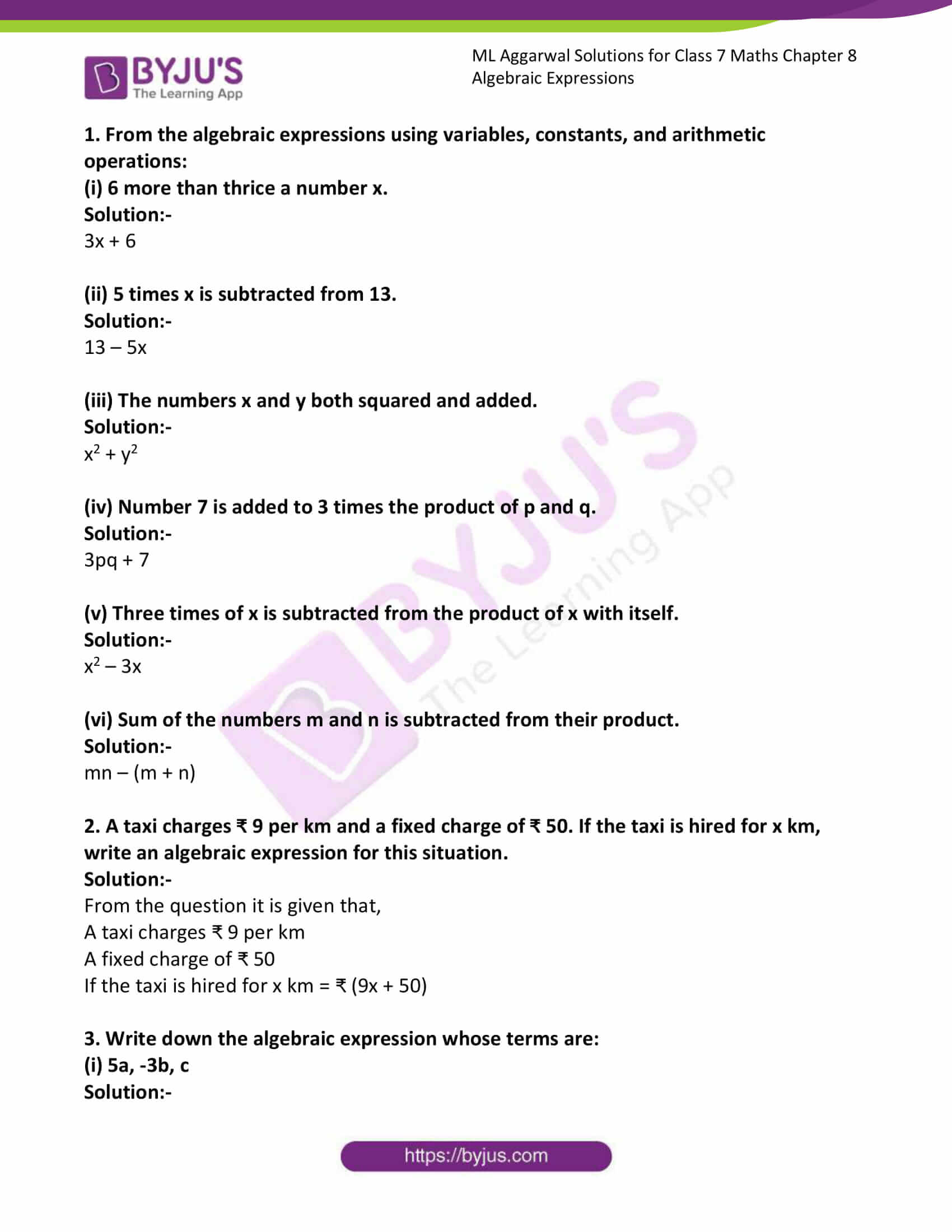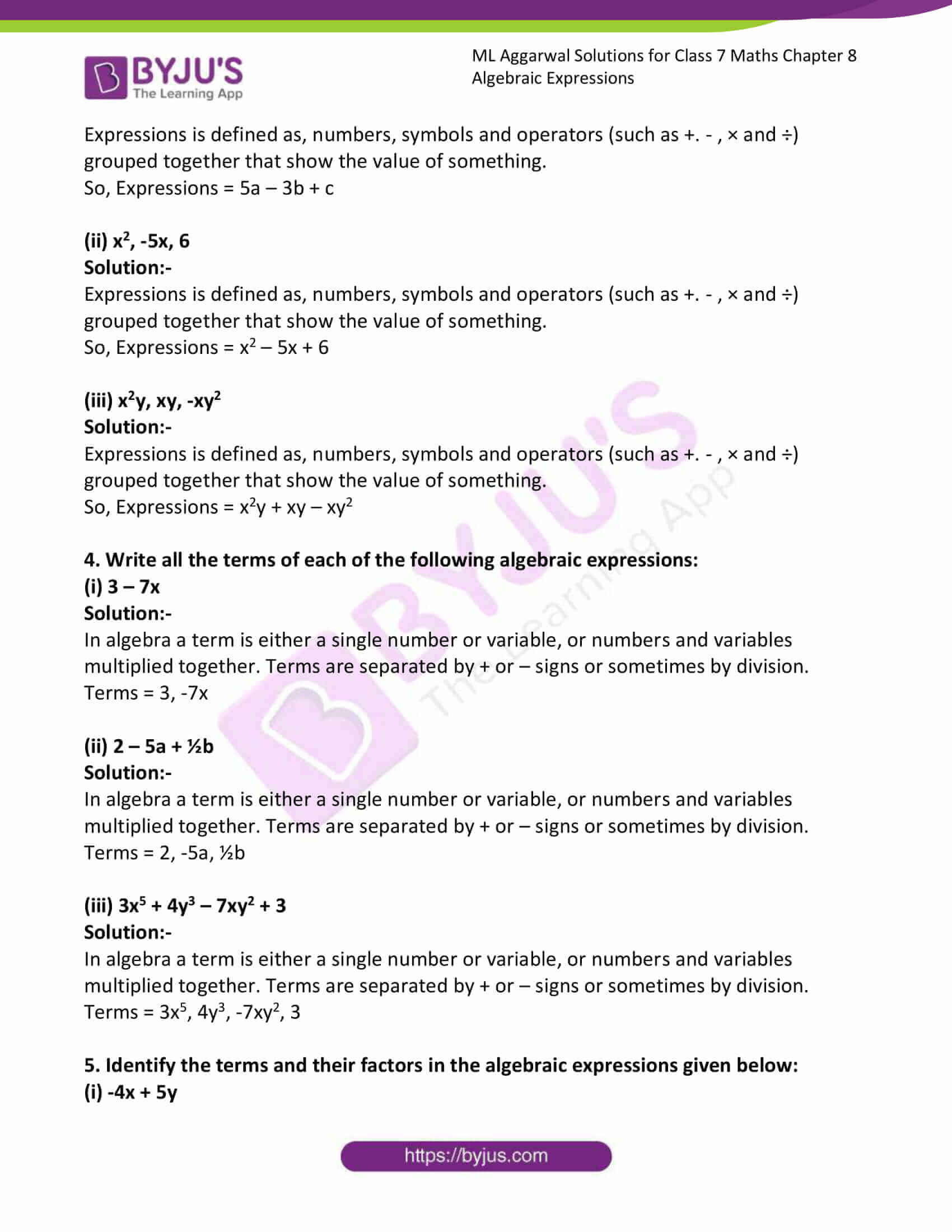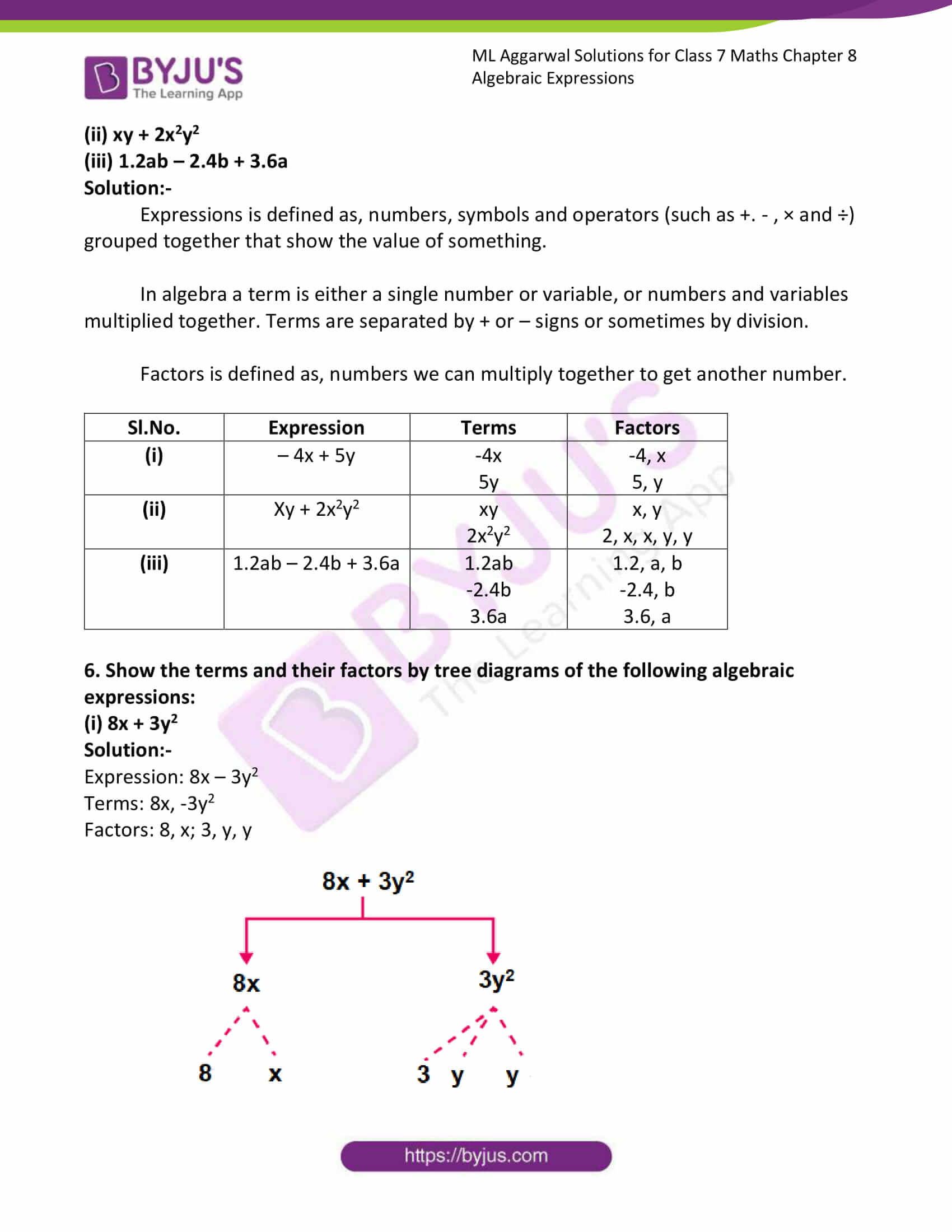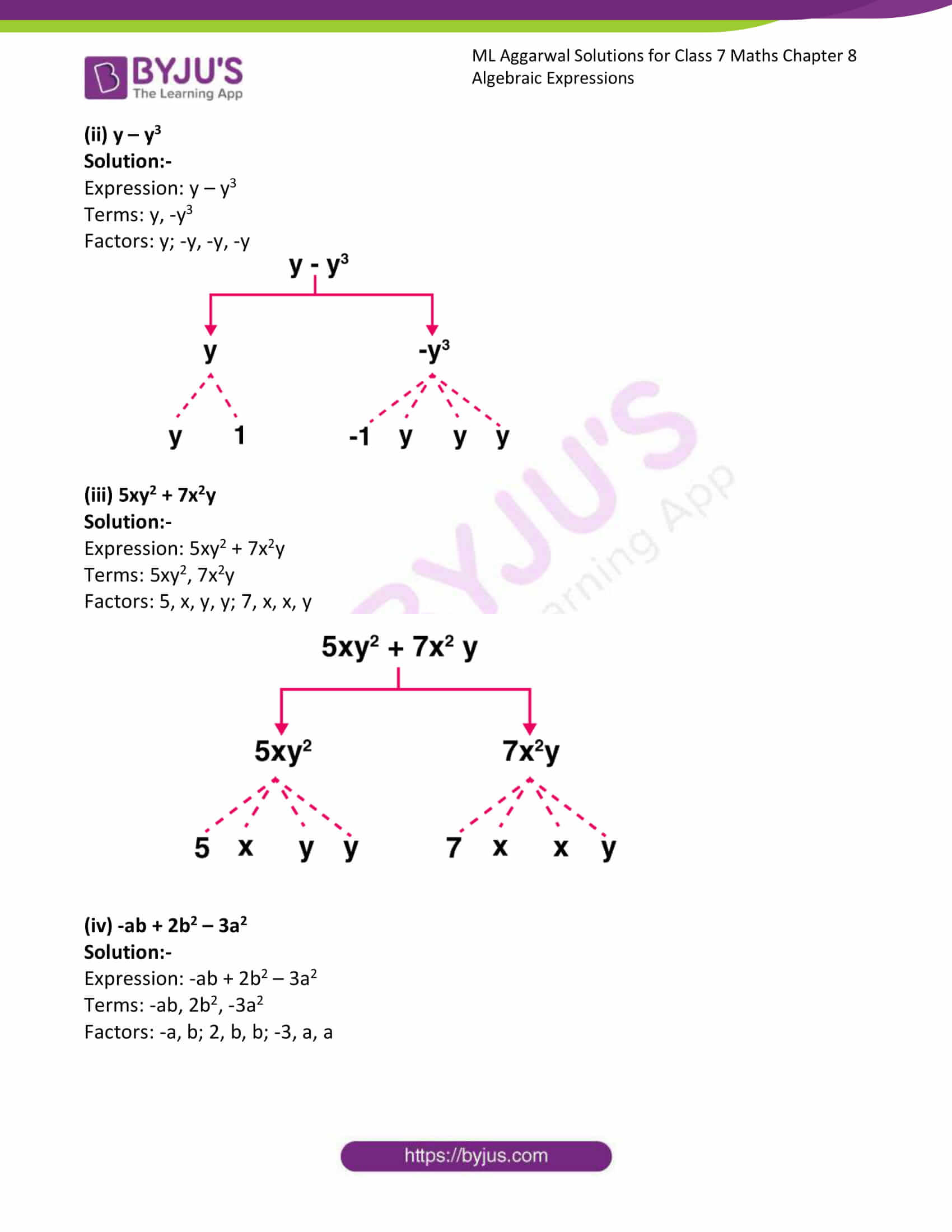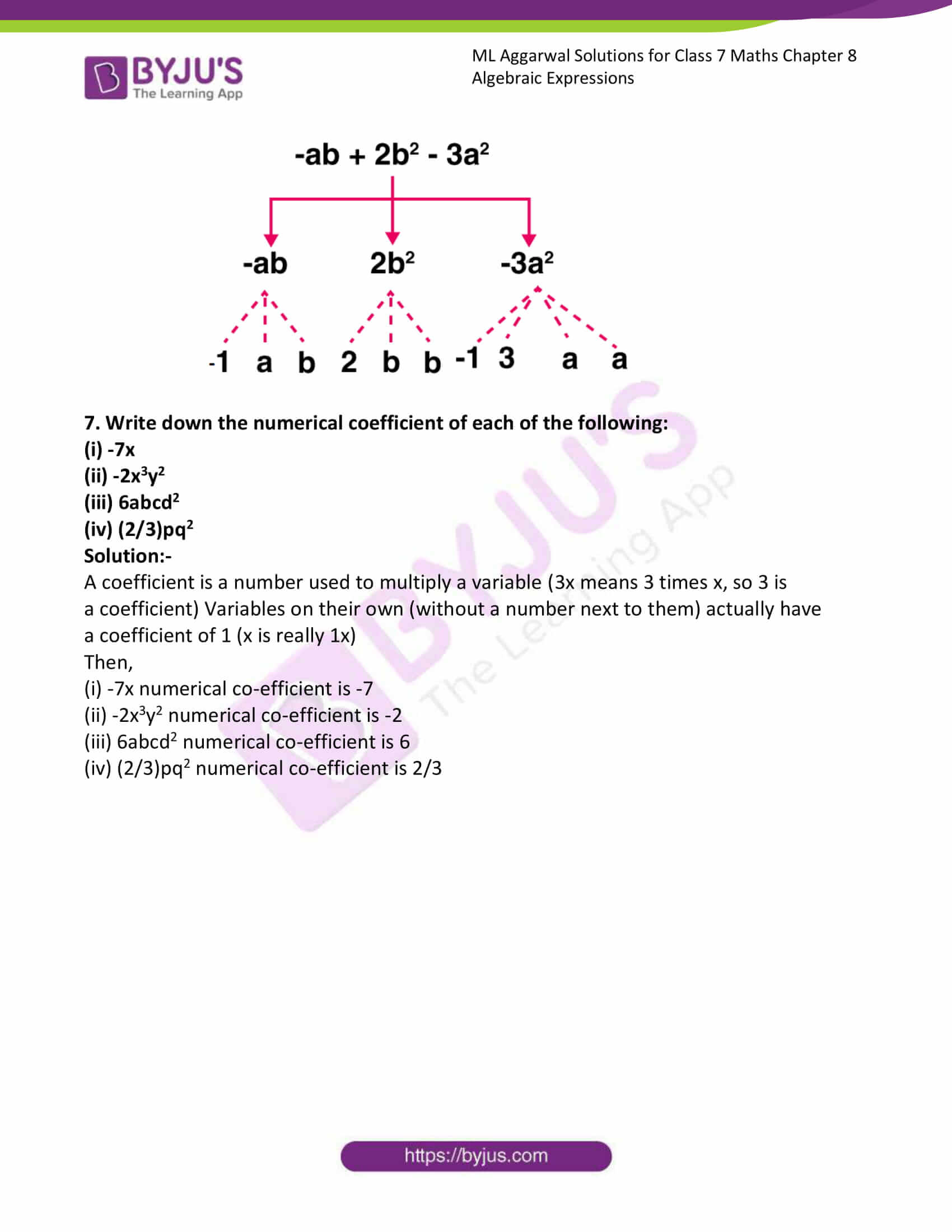### Access answers to ML Aggarwal Solutions for Class 7 Maths Chapter 8 Algebraic Expressions

1. From the algebraic expressions using variables, constants, and arithmetic operations:
(i) 6 more than thrice a number x.

Solution:-

3x + 6

(ii) 5 times x is subtracted from 13.

Solution:-

13 – 5x

(iii) The numbers x and y both squared and added.

Solution:-

x2 + y2

(iv) Number 7 is added to 3 times the product of p and q.

Solution:-

3pq + 7

(v) Three times of x is subtracted from the product of x with itself.

Solution:-

x2 – 3x

(vi) Sum of the numbers m and n is subtracted from their product.

Solution:-

mn – (m + n)

2. A taxi charges ₹ 9 per km and a fixed charge of ₹ 50. If the taxi is hired for x km, write an algebraic expression for this situation.

Solution:-

From the question it is given that,

A taxi charges ₹ 9 per km

A fixed charge of ₹ 50

If the taxi is hired for x km = ₹ (9x + 50)

3. Write down the algebraic expression whose terms are:
(i) 5a, -3b, c

Solution:-

Expressions is defined as, numbers, symbols and operators (such as +. – , × and ÷) grouped together that show the value of something.

So, Expressions = 5a – 3b + c

(ii) x2, -5x, 6

Solution:-

Expressions is defined as, numbers, symbols and operators (such as +. – , × and ÷) grouped together that show the value of something.

So, Expressions = x2 – 5x + 6

(iii) x2y, xy, -xy2

Solution:-

Expressions is defined as, numbers, symbols and operators (such as +. – , × and ÷) grouped together that show the value of something.

So, Expressions = x2y + xy – xy2

4. Write all the terms of each of the following algebraic expressions:

(i) 3 – 7x

Solution:-

In algebra a term is either a single number or variable, or numbers and variables multiplied together. Terms are separated by + or – signs or sometimes by division.

Terms = 3, -7x

(ii) 2 – 5a + ½b

Solution:-

In algebra a term is either a single number or variable, or numbers and variables multiplied together. Terms are separated by + or – signs or sometimes by division.

Terms = 2, -5a, ½b

(iii) 3x5 + 4y3 – 7xy2 + 3

Solution:-

In algebra a term is either a single number or variable, or numbers and variables multiplied together. Terms are separated by + or – signs or sometimes by division.

Terms = 3x5, 4y3, -7xy2, 3

5. Identify the terms and their factors in the algebraic expressions given below:
(i) -4x + 5y
(ii) xy + 2x2y2
(iii) 1.2ab – 2.4b + 3.6a

Solution:-

Expressions is defined as, numbers, symbols and operators (such as +. – , × and ÷) grouped together that show the value of something.

In algebra a term is either a single number or variable, or numbers and variables multiplied together. Terms are separated by + or – signs or sometimes by division.

Factors is defined as, numbers we can multiply together to get another number.

 Sl.No. Expression Terms Factors (i) – 4x + 5y -4x 5y -4, x 5, y (ii) Xy + 2x2y2 xy 2x2y2 x, y 2, x, x, y, y (iii) 1.2ab – 2.4b + 3.6a 1.2ab -2.4b 3.6a 1.2, a, b -2.4, b 3.6, a

6. Show the terms and their factors by tree diagrams of the following algebraic expressions:
(i) 8x + 3y2

Solution:-

Expression: 8x – 3y2

Terms: 8x, -3y2

Factors: 8, x; 3, y, y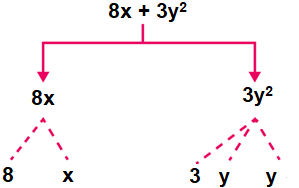(ii) y – y3

Solution:-

Expression: y – y3

Terms: y, -y3

Factors: y; -y, -y, -y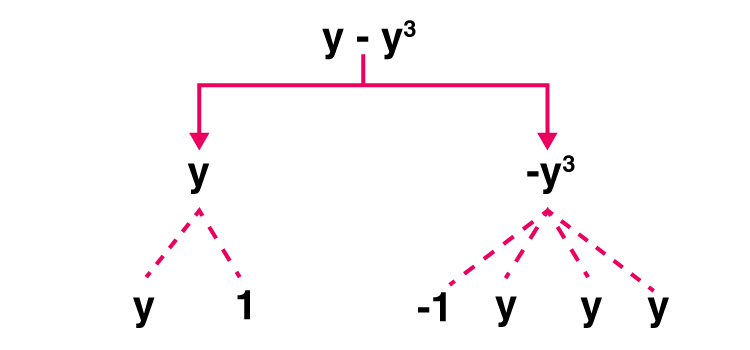(iii) 5xy2 + 7x2y

Solution:-

Expression: 5xy2 + 7x2y

Terms: 5xy2, 7x2y

Factors: 5, x, y, y; 7, x, x, y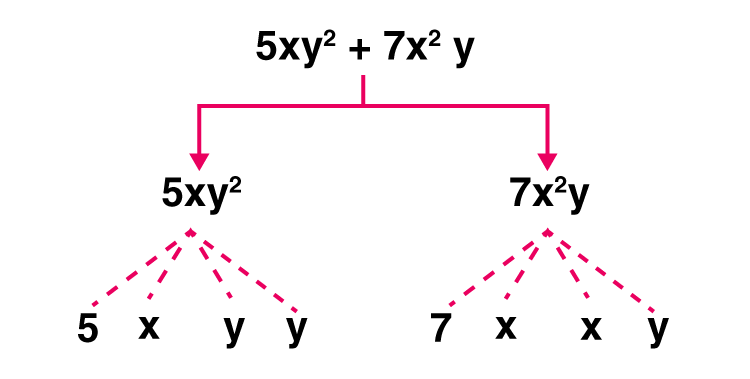(iv) -ab + 2b2 – 3a2

Solution:-

Expression: -ab + 2b2 – 3a2

Terms: -ab, 2b2, -3a2

Factors: -a, b; 2, b, b; -3, a, a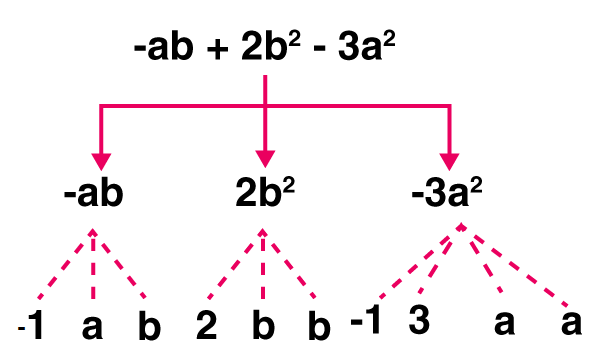7. Write down the numerical coefficient of each of the following:
(i) -7x
(ii) -2x3y2
(iii) 6abcd2

(iv) (2/3)pq2

Solution:-

A coefficient is a number used to multiply a variable (3x means 3 times x, so 3 is a coefficient) Variables on their own (without a number next to them) actually have a coefficient of 1 (x is really 1x)

Then,

(i) -7x numerical co-efficient is -7

(ii) -2x3y2 numerical co-efficient is -2

(iii) 6abcd2 numerical co-efficient is 6

(iv) (2/3)pq2 numerical co-efficient is 2/3